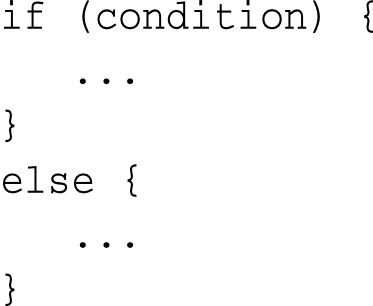# 5.2 - else 陳述

else 如同英文字義，否則的意思，通常放在 if 的後面，若 if 的條件為真，就執行 if 底下大括弧圍起來的程式區塊，若為假，就執行 else 底下大括弧圍起來的程式區塊。``````#include <iostream>

int main() {
char a = 'h';
char b = 'k';

if (a == b) {
std::cout << "Welcome to my world!" << std::endl;
}
else {
std::cout << "How do you do?"<< std::endl;
}

return 0;
}

/* 《程式語言教學誌》的範例程式
http://kaiching.org/
檔名：u0502_1.cpp
功能：示範 C++ 的 else 陳述
作者：張凱慶*/``````

 \$ g++ u0502_1.cpp \$ ./a.out How do you do? \$

``if (a == b) {``

``````else {
std::cout << "How do you do?"<< std::endl;
}``````

if-else 也可以是巢狀 (nested) 的使用，例如

``````#include <iostream>

int main() {
char a = 'h';
char b = 'k';

if (a == 'a') {
std::cout << "Yes!" << std::endl;
}
else {
if (a == b) {
std::cout << "No!" << std::endl;
}
else {
std::cout << "What?" << std::endl;
}
}

return 0;
}

/* 《程式語言教學誌》的範例程式
http://kaiching.org/
檔名：u0502_2.cpp
功能：示範 C++ 的 else 陳述
作者：張凱慶*/``````

 \$ g++ u0502_2.cpp \$ ./a.out What? \$

``````if (a == b) {
std::cout << "No!" << std::endl;
}
else {
std::cout << "What?" << std::endl;
}``````

``````if (a == 'a') {
std::cout << "Yes!" << std::endl;
}
else if (a == b) {
std::cout << "No!" << std::endl;
}
else {
std::cout << "What?" << std::endl;
}``````